Music Basics Quiz
Last Name *
How long is this note/rest in 4/4?
1 point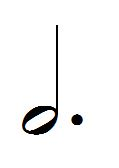What type of note/rest(s) is (are) these?
1 point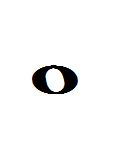Name this note
1 point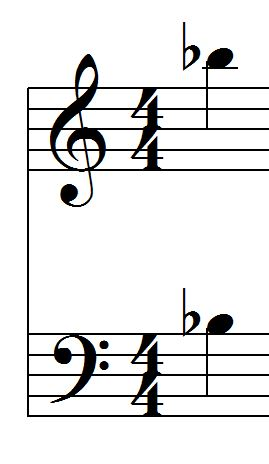What accidental is this?
1 point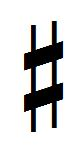What type of clef is this?
1 point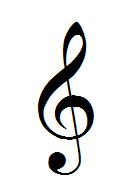Name this note
1 point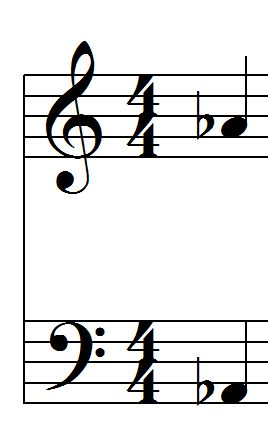Name this note
1 point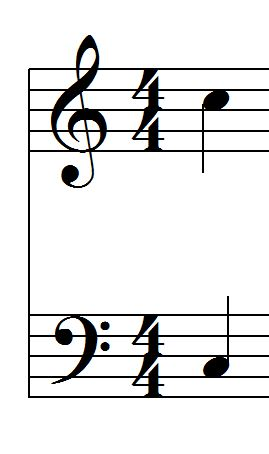First Name *
What type of clef is this?
1 point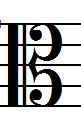How long is this note/rest in 4/4?
1 point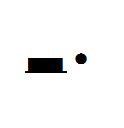What type of note/rest(s) is (are) these?
1 point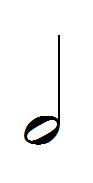Name this note
1 point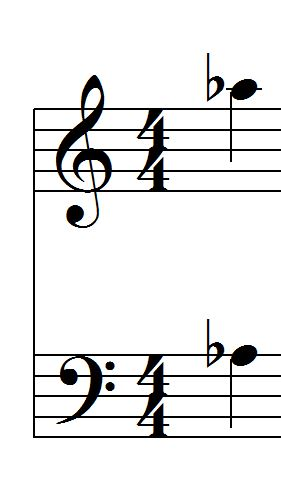What accidental is this?
1 point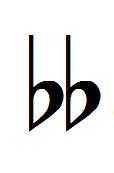Name this note
1 point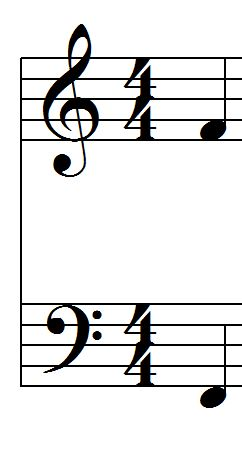How long is this note/rest in 4/4?
1 point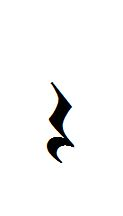What type of note/rest(s) is (are) these?
1 point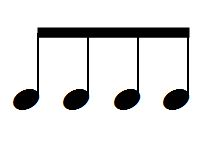What type of note/rest(s) is (are) these?
1 point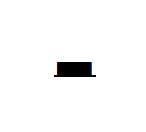How long is this note/rest in 4/4?
1 pointName this note
1 point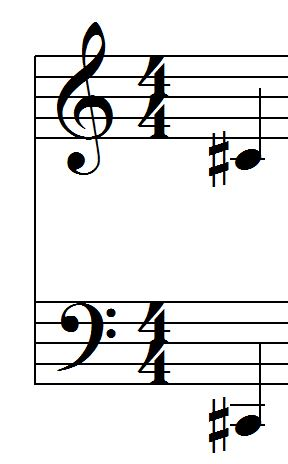What type of note/rest(s) is (are) these?
1 point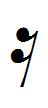Name this note
1 point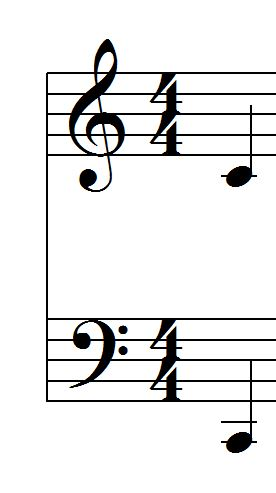What type of note/rest(s) is (are) these?
1 point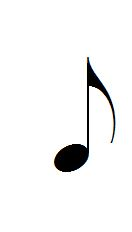What accidental is this?
1 point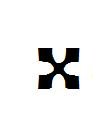What type of note/rest(s) is (are) these?
1 point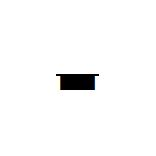Name this note
1 point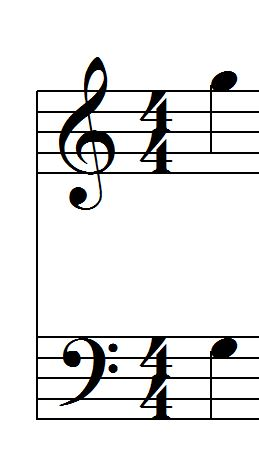What type of note/rest(s) is (are) these?
1 point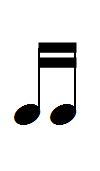How long is this note/rest in 4/4?
1 pointName this note
1 pointName this note
1 point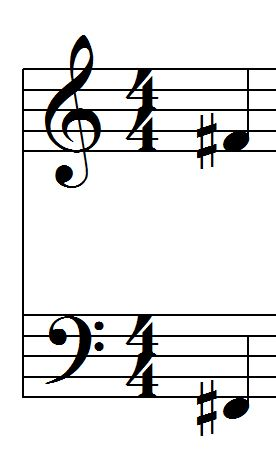Name this note
1 point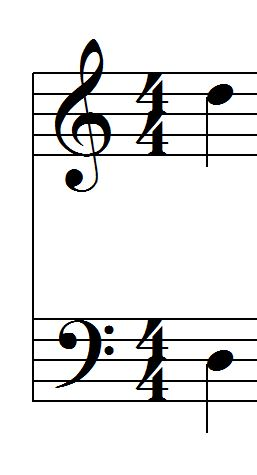What accidental is this?
1 point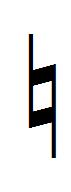How long is this note/rest in 4/4?
1 point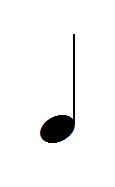Name this note
0 points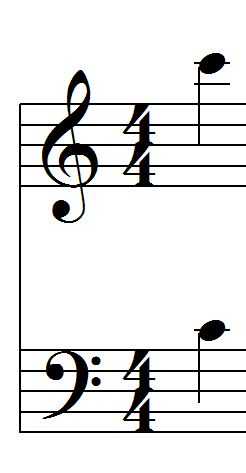What type of note/rest(s) is (are) these?
1 point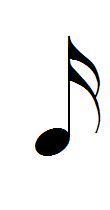What accidental is this?
1 point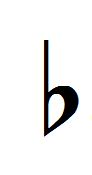Name this note
1 point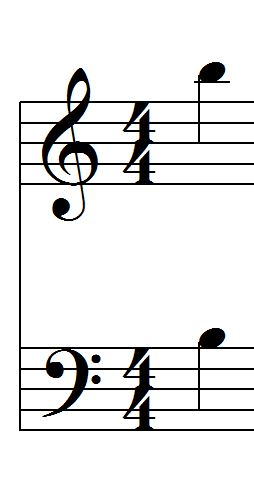What type of clef is this?
1 point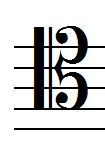Name this note
1 point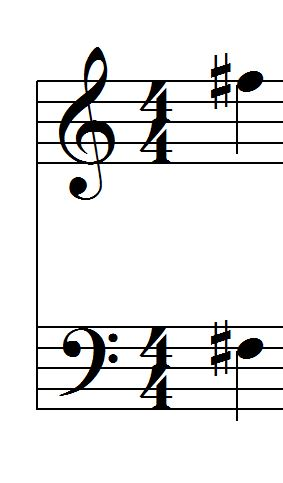How long is this note/rest in 4/4?
1 pointName this note
1 point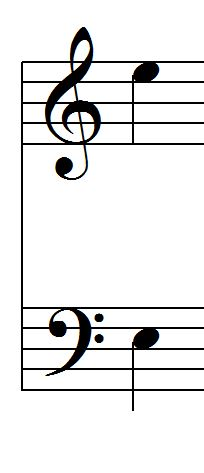What type of note/rest(s) is (are) these?
1 point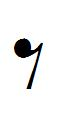Name this note
1 point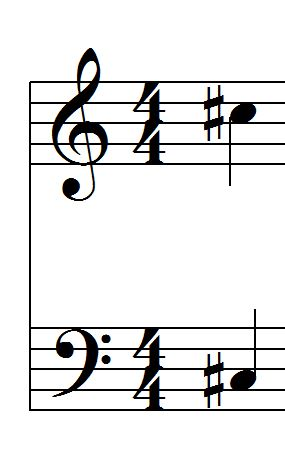How long is this note/rest in 4/4?
1 pointHow long is this note/rest in 4/4?
1 point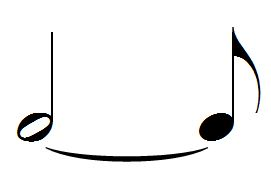Name this note
1 point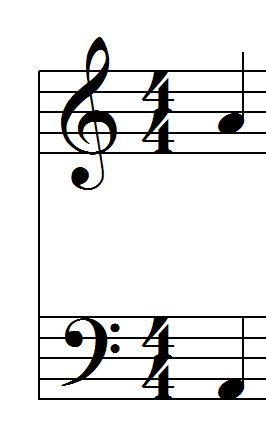What type of note/rest(s) is (are) these?
1 pointHow long is this note/rest in 4/4?
0 points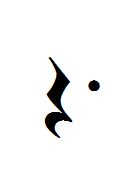How long is this note/rest in 4/4?
1 point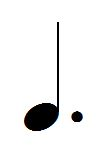Name this note
1 point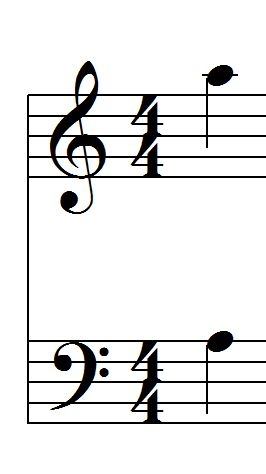What type of clef is this?
1 point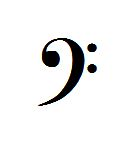Name this note
1 point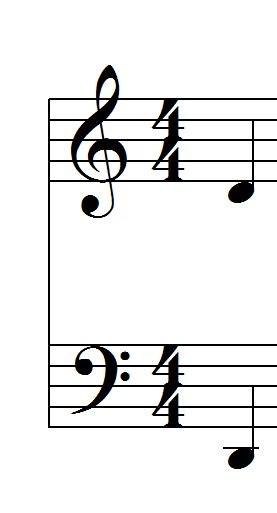Name this note
1 point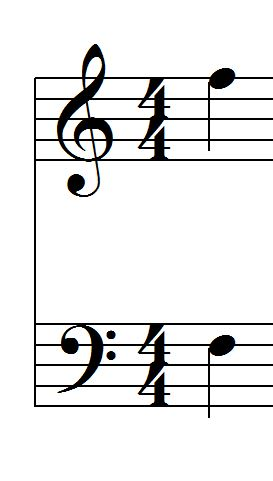Name this note
1 point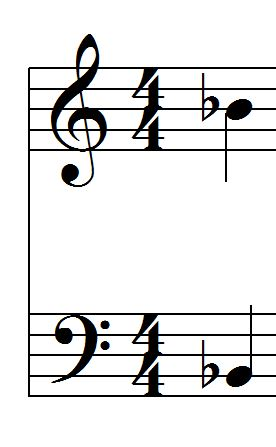How long is this note/rest in 4/4?
1 pointName this note
1 point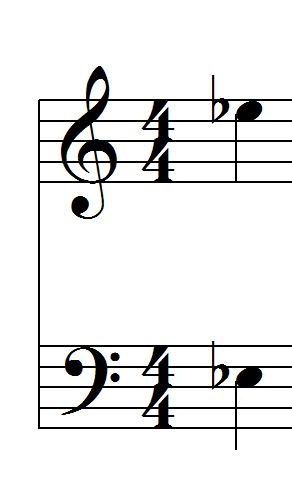Name this note
1 point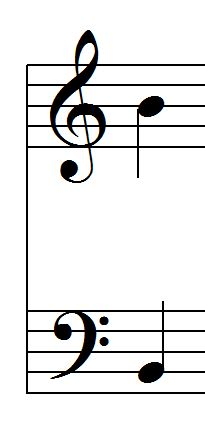Name this note
1 pointWhat type of note/rest(s) is (are) these?
1 pointHow long is this note/rest in 4/4?
1 point